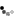CALYPSO Real-Time Sea Experiment 2019

Alboran Sea – March-April 2019

 P.F.J. Lermusiaux, P.J. Haley, C. Mirabito, M. Doshi, A. Gkirgkis, W.H. Ali, C. Kulkarni, A. Gupta Massachusetts Institute of Technology Center for Ocean Engineering Mechanical Engineering Cambridge, MassachusettsMSEAS Deterministic Ocean Forecasts MSEAS Probabilistic Ocean Forecasts MSEAS Methods & Systems Atmos. Forecasts Results From Partners Data sources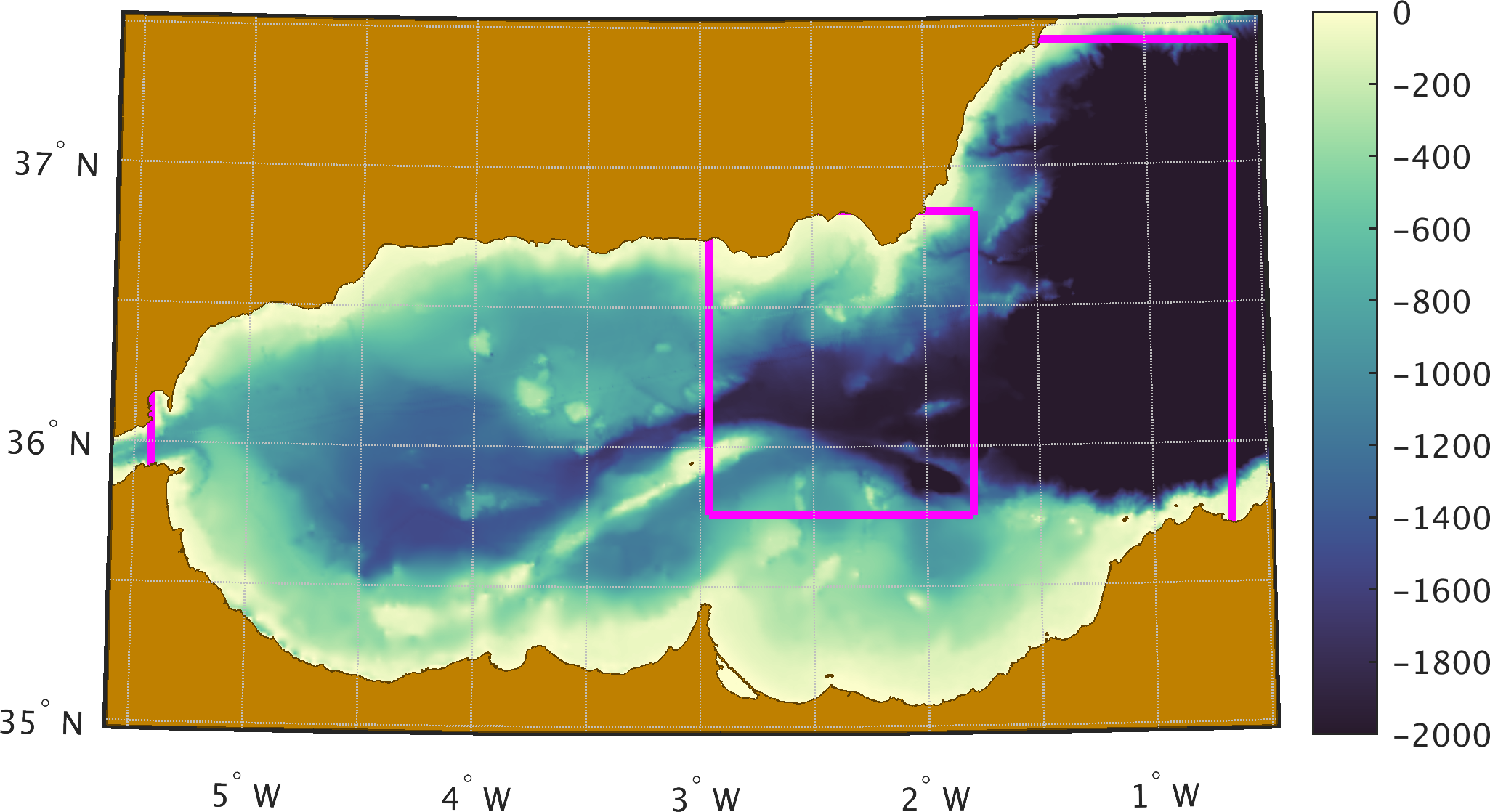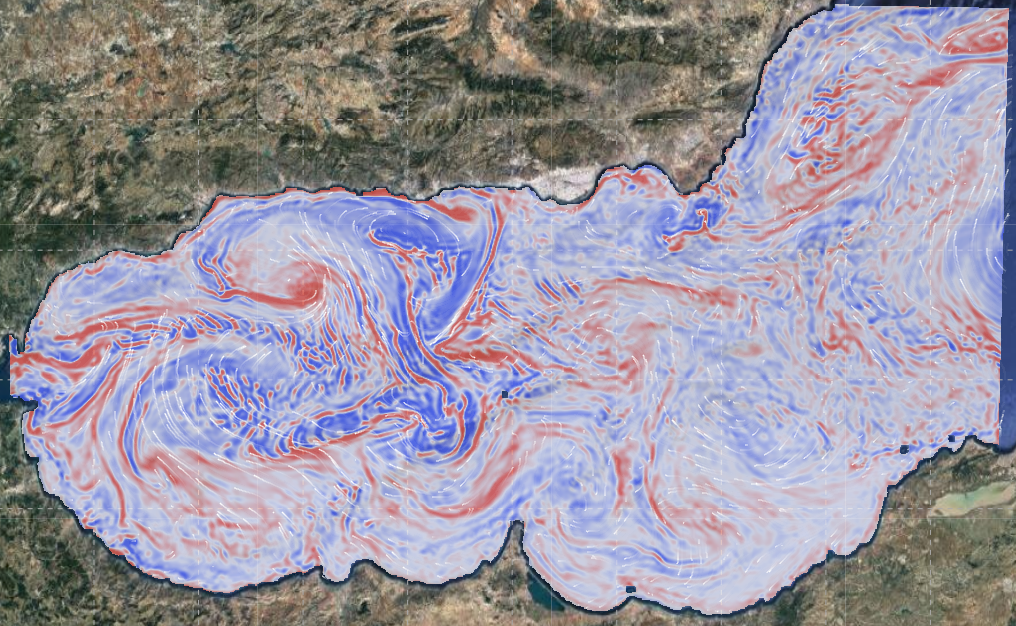The CALYPSO 2019 experiment occurs in the Alboran Sea from March 27 to April 11, 2019. Our specific objectives are to: (i) Utilize our new Lagrangian transport theory and methods to forecast, characterize and quantify ocean processes involved in the three-dimensional transports and transformation of water masses and subduction dynamics in the Alboran Sea; (ii) Apply and expand our multi-resolution submesoscale-to-regional-scale ocean modeling, 2-way nesting, and uncertainty predictions, for real-time forecasting and process studies; (iii) Help design field experiments and predict sampling strategies that maximize information on 4D pathways and dynamics in the region. We thank all of the CALYPSO team members for their inputs and collaboration. We also thank NCEP for their atmospheric forcing data.

This research is sponsored by the Office of Naval Research

## Real-time MSEAS Forecasting

• MSEAS Deterministic Ocean Forecasts  Real-Time Estimates of Present (15 Apr 0000Z) Environmental Conditions (downscaled from WMOP) Full Modeling Domain (1/200° resolution) Section along 4.25°W (1/200° resolution) Interactive Ocean Physics Forecast 3D Lagrangian Fct.of Subduction Interactive 96-hrFlow Map Forecast 0m Temperature 2m Vorticity Temperature Salinity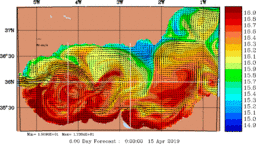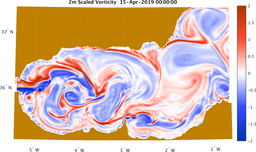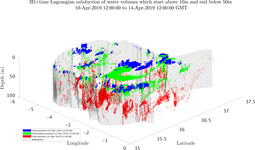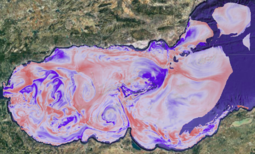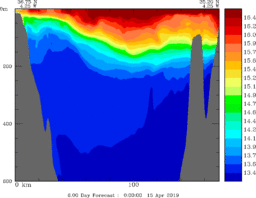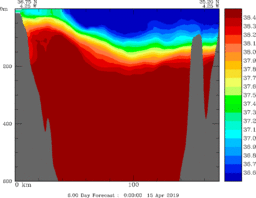MSEAS high-resolution tidally-forced forecasts downscaled from WMOP
 Nowcast and Forecast Productswith dynamics descriptions Analyses and Forecasts Issued On March 2019 April 2019 25 26 27 28 29 30 31 1 2 3 4 5 6 7 8 9 10 Ocean Physics Horizontal Maps Central Forecast X X X X X X X X X X X X X X X X X Interactive Forecast X X X X X X X X X X X X X X X X X Vertical Sections Central Forecast X X X X X X X X X X X X X X X X X Flow Maps Forward flowmaps display at the initial location of a water parcel the final position of that water parcel as it is advected over a specified time window. For instance, if the value of the 96 hour forward z-flowmap at a given (x, y, z) location is 50m, the final depth of that point will be 50m after 96 hours of being advected in the flow. Hence, the z-flowmap for example does not indicate where the water parcel goes down nor where it ends up. Interactive Forecast 6-hour X X X X X X X X X X X X X X X X X 96-hour X X X X X X X X X X X X X X X X X Lagrangian Coherent Structures The figures in this row show the finite-time Lyapunov exponents (FTLEs) in the domain, at different times. These FTLE computations are for a time window of 6 or 96 hours for the forecasts on various dates as specified in the figures captions. The flowmaps were calculated using a PDE-based approach with 5th order spatial and 3rd order temporal schemes, and a novel composition based approach. The FTLE field specifies the amount of stretching of the flowmap in the considered time interval. Specifically, the forward / backward FTLE is a logarithmic scaling of the dominant singular values of the forward / backward flowmap with the time duration of interest. The forward FTLE ridges thus highlight codimension-one manifolds (curves in 2D, surfaces in 3D) that repel particles starting on either side, whereas the backward FTLE ridges highlight codimension-one manifolds that attract particles towards the ridge that originally start far apart. As a reminder, 12:00Z = 13:00 CET, i.e., CET is 1 hour ahead of Zulu time. LCS Maps X X X X X X X X X X X X X X X X X Drifters The figures in this row of the table show the trajectories in the domain for a given time interval. For this project, the drifters are released at every 10 grid points, and the grid has a model resolution of 1/200 deg. These trajectory computations correspond to time windows of 48 and 72 hours, for the forecasts specified in the headers of the respective columns. Trajectories (Surface) 48-hour X X X X X X X X X X X X X X X X X 72-hour X X X X X X X X X X X X X X X X X Special Lagrangian Products Special products, commonly still images, of our MSEAS forecasts of the ocean physics and Lagrangian 3D transport of waters (e.g. waters starting at various depths). What is plotted is the depth reached by the water parcel by the end of the forecast, starting on a specific date and time at that (x, y, z) location (i.e., it does not show the horizontal displacement of the parcel, only the depth reach by a parcel released at x, y, z). For the 3D+time Lagrangian subduction of waters, what is shown are the motions in 3D of water volumes that subduct the most from the surface layers to deeper layers (computed based on our forward flowmap forecast). 96-hour forward flow map still images X X X X X X X X X X X X X X X X X 3D Lagrangian subduction of waters X X X X X X X X X X X X X X X

MSEAS high-resolution tidally-forced forecasts downscaled from HYCOM
 Nowcast and Forecast Productswith dynamics descriptions Analyses and Forecasts Issued On Feb. 2019 March 2019 April 2019 25 27 5 6 11 23 25 30 31 1 2 3 4 5 6 7 8 9 10 Ocean Physics Horizontal Maps Central Forecast X X X X X X X X X X X X X X X X X X X Interactive Forecast X X X X X X X X X X X X X X X X X X X Vertical Sections Central Forecast X X X X X X X X X X X X X X X X X X X Flow Maps Forward flowmaps display at the initial location of a water parcel the final position of that water parcel as it is advected over a specified time window. For instance, if the value of the 96 hour forward z-flowmap at a given (x, y, z) location is 50m, the final depth of that point will be 50m after 96 hours of being advected in the flow. Hence, the z-flowmap for example does not indicate where the water parcel goes down nor where it ends up. Interactive Forecast 6-hour X X X X X X X X X X X X X X X X X X X 96-hour X X X X X X X X X X X X X X X X X X X Lagrangian Coherent Structures The figures in this row show the finite-time Lyapunov exponents (FTLEs) in the domain, at different times. These FTLE computations are for a time window of 6 or 96 hours for the forecasts on various dates as specified in the figures captions. The flowmaps were calculated using a PDE-based approach with 5th order spatial and 3rd order temporal schemes, and a novel composition based approach. The FTLE field specifies the amount of stretching of the flowmap in the considered time interval. Specifically, the forward / backward FTLE is a logarithmic scaling of the dominant singular values of the forward / backward flowmap with the time duration of interest. The forward FTLE ridges thus highlight codimension-one manifolds (curves in 2D, surfaces in 3D) that repel particles starting on either side, whereas the backward FTLE ridges highlight codimension-one manifolds that attract particles towards the ridge that originally start far apart. As a reminder, 12:00Z = 13:00 CET, i.e., CET is 1 hour ahead of Zulu time. LCS Maps X X X X X X X X X X X X X X Drifters The figures in this row of the table show the trajectories in the domain for a given time interval. For this project, the drifters are released at every 10 grid points, and the grid has a model resolution of 1/200 deg. These trajectory computations correspond to time windows of 48 and 72 hours, for the forecasts specified in the headers of the respective columns. Trajectories (Surface) 48-hour X X X X X X X X X X X X X X 72-hour X X X X X X X X X X X X X X Special Lagrangian Products Special products, commonly still images, of our MSEAS forecasts of the ocean physics and Lagrangian 3D transport of waters (e.g. waters starting at various depths). What is plotted is the depth reached by the water parcel by the end of the forecast, starting on a specific date and time at that (x, y, z) location (i.e., it does not show the horizontal displacement of the parcel, only the depth reach by a parcel released at x, y, z). For the 3D+time Lagrangian subduction of waters, what is shown are the motions in 3D of water volumes that subduct the most from the surface layers to deeper layers (computed based on our forward flowmap forecast). 96-hour forward flow map still images X X X X X X X X X X X X X X X 3D Lagrangian subduction of waters X X X X X X X X X X X

MSEAS high-resolution tidally-forced forecasts downscaled from CMEMS
 Nowcast and Forecast Productswith dynamics descriptions Analyses and Forecasts Issued On March 2019 April 2019 26 30 31 1 2 3 4 5 6 7 8 9 10 Ocean Physics Horizontal Maps Central Forecast X X X X X X X X X X X X X Interactive Forecast X X X X X X X X X X X X X Vertical Sections Central Forecast X X X X X X X X X X X X X
•

• MSEAS Probabilistic Ocean Forecasts: Physics-balanced stochastic models and ESSE dominant subspace decompositions are used to represent the dominant uncertainties in the initial and boundary conditions for T, S, u, v, w and eta fields for each of the WMOP, HYCOM and CMEMS modeling system fields, in the atmospheric surface forcing flux fields, and in the tidal forcing parameters.  Nowcast and Forecast Uncertainty Productswith dynamics descriptions Probabilistic Analyses and Forecasts Issued On April 2019 3 6 8 10 ProbabilisticOcean Physics Horizontal Maps Mean and Std. Dev. X X X X Interactive Mean and Std. Dev. Forecast X X X X Vertical Sections Mean and Std. Dev. X X X X
•

• Methods and Systems: The probabilistic MIT-MSEAS Primitive-Equation (PE) ocean modeling system is utilized in real-time to provide ocean forecasts for Lagrangian transport and analyses in the region. The modeling system was set-up in an implicit 2-way nesting configuration (1/200° resolution Alboran Sea domain and 1/600° resolution process domains). The ocean forecasts are initialized from either HYCOM or WMOP or CMEMS, downscaled to higher resolution and updated with ocean data from varied open sources of opportunity (CTDs, ARGO floats, gliders, SST [AVHRR-METOP satellite data from OSI SAF], etc.). Ensemble forecasts are initialized using ESSE procedures. These ocean simulations are forced by atmospheric flux fields forecast by the Global Forecast System (GFS) 0.25° model from the National Centers for Environmental Prediction (NCEP) and by tidal forcing from TPXO8, but adapted to the high-resolution bathymetry and coastlines.
•

• MSEAS-processed atmospheric forcing flux forecasts:
NCEP GFS 0.25° February 2019 March 2019
Daily average wind stress, E-P, heat flux, and SW Radiation (from 0Z forecast each day)
Flux snapshot plots (a single 60hr fct issued daily) X X X X X X X X X X X X X X X X X X X X X X
Forecast snapshot plots (Four 6-hour forecasts/day) 22 23 24 25 26 27 28 1 2 3 4 5 6 7 8 9 10 11 12 13 14 15
NCEP GFS 0.25° March 2019
Daily average wind stress, E-P, heat flux, and SW Radiation (from 0Z forecast each day)
Flux snapshot plots (a single 60hr fct issued daily) X X X X X X X X X X X X X X X X
Forecast snapshot plots (Four 6-hour forecasts/day) 16 17 18 19 20 21 22 23 24 25 26 27 28 29 30 31
NCEP GFS 0.25° April 2019
Daily average wind stress, E-P, heat flux, and SW Radiation (from 0Z forecast each day)
Flux snapshot plots (a single 144hr fct issued daily) X X X X X X X X X X
Forecast snapshot plots (Four 6-hour forecasts/day) 1 2 3 4 5 6 7 8 9 10
•

• MSEAS plots of SST Images
•

• Results from our CALYPSO partners:
•

• Acknowledgements: We thank our CALYPSO colleagues for their inputs and collaboration. We especially thank Baptiste Mourre and the SOCIB team for the WMOP model fields and data. We also thank Uwe Send for his mooring data, and Tamay Özgökmen and Pierre Poulain for their drifter data. We also thank Emanuela Clementi, the Centro Euro-Mediterraneo sui Cambiamenti Climatici (CMCC), and Nadia Pinardi for the CMEMS data in the Mediterranean. We also wish to thank Matthew Pyle, Eric Rogers, Geoff DiMego, and Arun Chawla of NCEP for help and support for atmospheric forcing data.

 Top of page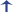## Data sources

 Top of page